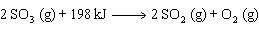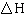Name:    Practice Test 2A

Multiple Choice

1.

It has been shown experimentally that a beam of electrons can be diffracted.  This is evidence that electrons
 A) are lighter than protons B) have wave properties C) have particle properties D) are negative

2.

Which of the following statements is NOT true?
 A) The Heisenberg uncertainty principle states that it is impossible to determine accurately both the position and velocity of an electron. B) Rutherford’s alpha particle scattering experiment demonstrated that most of the mass of an atom is in a small dense nucleus. C) The solution of Schrodinger’s wave equation permits you to predict the exact location and momentum of a hydrogen atom’s electron at any time. D) de Broglie reasoned that matter could have both wave and particle properties.

3.

Which of the following statements concerning the reaction is correct?  Assume constant pressure.A) The enthalpy of products exceeds that of the reactants B) The reaction is exothermic C) The sign offor the reaction is negative D) The enthalpy of reactants exceeds that of the products

4.

A chemical system produces 155 kJ of heat and does 22 kJ of work.  What is DE for the surroundings?
 A) 177 kJ B) -133 kJ C) 133 kJ D) -177 kJ

5.

Calculate the frequency of an electron travelling at 1.85x107 m/s. (mass of electron = 9.11x10-28 g)
 A) 1.31x10-19 s-1 B) 1.18x10-2 s-1 C) 7.63x1018 s-1 D) 3.93x10-11 s-1

6.

When a 3.80 g sample of liquid octane (C8H18) is burned in a bomb calorimeter, the temperature of the calorimeter rises by 27.3 °C.  Determine the DH for this reaction in kJ/mol octane.  (Heat capacity of the calorimeter is 6.18 kJ/°C)
 A) -16.7x103 kJ/mol B) 5.07x103 kJ/mol C) -5.07x103 kJ/mol D) -44.4x103 kJ/mol

7.

How much energy (in J) is contained in 1.00 mol of 552 nm photons?
 A) 3.60x10-19 J B) 2.17x105 J C) 5.98x10-43 J D) 3.60x10-28 J

8.

If the same amount of heat is added to 50 g or each metal shown below, which will attain the highest temperature?
 A) chromium (0.447 J/g °C) B) cobalt (0.418 J/g °C) C) gold (0.129 J/g °C) D) copper (0.385 J/g °C)

9.

Blue light has a _________ frequency and a __________wavelength than red light.
 A) lower, longer B) higher, longer C) higher, shorter D) lower, shorter

10.

The angular momentum quantum number (l) is best associated with the
 A) number of orbitals in a sub-level. B) orientation in space of an orbital C) energy of the orbital. D) shape of an orbital.

11.

Hydrogen gas reacts with oxygen to form water.

2 H2 (g)  +  O2 (g)  ®   2 H2O (g)               DH = ???

In one experiment 1.888 g of hydrogen react to form 226.4 kJ of heat.  Based on this information, calculate the enthalpy for the reaction as written above.
 A) -241.7 kJ B) +241.7 kJ C) -483.5 kJ D) -212.0 kJ

12.

Which of the following combination of quantum numbers is allowed?
 A) n =3; l =2; ml = +3; ms= +1/2 B) n =3; l =3; ml = +1; ms= –1/2 C) n =4; l =3; ml = 0; ms= -1/2 D) n =3; l =2; ml = –1; ms= –1

13.

Which of the following correctly lists the electromagnetic waves in order of decreasing energy?
 A) microwaves > ultraviolet > infrared > x-rays B) microwaves > infrared > ultraviolet > x-rays C) x-rays > microwaves > infrared > ultraviolet D) x-rays > ultraviolet > infrared > microwaves

14.

All the following statements are TRUE, except
 A) heat flows from the system into the surroundings in an exothermic process. B) heat flows from the system into the surroundings in an endothermic process. C) the value of q is positive in an endothermic process D) the value of q is positive when heat flows into the system from the surroundings.

15.

What is the wavelength of the radiation emitted when an electron undergoes a transition from n = 5 to n = 3?
 A) 1282 nm B) 384.6 nm C) 683.8 nm D) 205.1 nm